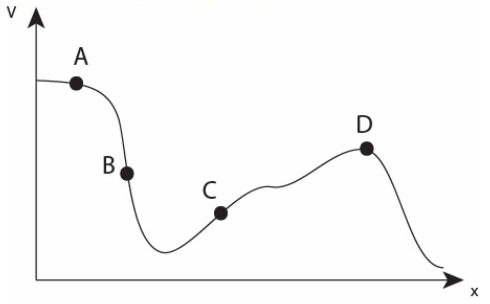# Problem: The electric potential in a certain region is plotted in the following graph1. At which point is the magnitude of the E-field greatest?2. At which point is the direction of the E-field along the negative x-axis?

###### FREE Expert Solution

The electric field is given by:

$\overline{){\mathbf{E}}{\mathbf{=}}{\mathbf{-}}\frac{\mathbf{d}\mathbf{V}}{\mathbf{d}\mathbf{x}}}$

The electric field is the slope of the curve.

(1)

The magnitude of the electric field is greatest where the slope is greatest.

The slope is greatest at point B.

92% (60 ratings)###### Problem Details

The electric potential in a certain region is plotted in the following graph1. At which point is the magnitude of the E-field greatest?

2. At which point is the direction of the E-field along the negative x-axis?